# Visualize bundles and metrics on bundles¶

First, let’s download some available datasets. Here we are using a dataset which provides metrics and bundles.

```import numpy as np
from fury import window, actor
from dipy.tracking.streamline import transform_streamlines, length

interactive = False  # set to True to show the interactive display window

fetch_bundles_2_subjects()
bundles=['cg.left', 'cst.right'])
```

Out:

```Data size is approximately 234MB
Dataset is already in place. If you want to fetch it again please first remove the folder /Users/koudoro/.dipy/exp_bundles_and_maps
```

Store fractional anisotropy.

```fa = dix['fa']
```

Store grid to world transformation matrix.

```affine = dix['affine']
```

Store the cingulum bundle. A bundle is a list of streamlines.

```bundle = dix['cg.left']
```

It happened that this bundle is in world coordinates and therefore we need to transform it into native image coordinates so that it is in the same coordinate space as the `fa` image.

```bundle_native = transform_streamlines(bundle, np.linalg.inv(affine))
```

## Show every streamline with an orientation color¶

This is the default option when you are using `line` or `streamtube`.

```scene = window.Scene()

stream_actor = actor.line(bundle_native)

scene.set_camera(position=(-176.42, 118.52, 128.20),
focal_point=(113.30, 128.31, 76.56),
view_up=(0.18, 0.00, 0.98))

if interactive:
window.show(scene, size=(600, 600), reset_camera=False)

window.record(scene, out_path='bundle1.png', size=(600, 600))
```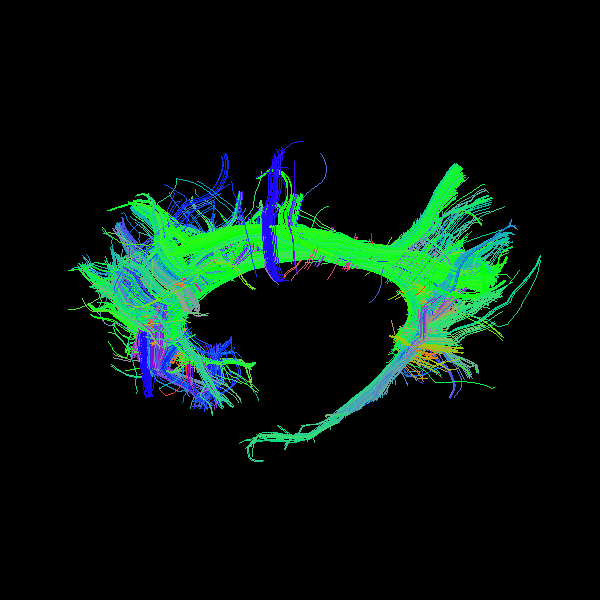You may wonder how we knew how to set the camera. This is very easy. You just need to run `window.show` once see how you want to see the object and then close the window and call the `camera_info` method which prints the position, focal point and view up vectors of the camera.

```scene.camera_info()
```

Out:

```# Active Camera
Position (-237.76, 115.97, 138.55)
Focal Point (112.80, 127.81, 76.06)
View Up (0.18, 0.00, 0.98)
```

## Show every point with a value from a volume with default colormap¶

Here we will need to input the `fa` map in `streamtube` or `line`.

```scene.clear()
stream_actor2 = actor.line(bundle_native, fa, linewidth=0.1)
```

We can also show the scalar bar.

```bar = actor.scalar_bar()

if interactive:
window.show(scene, size=(600, 600), reset_camera=False)

window.record(scene, out_path='bundle2.png', size=(600, 600))
```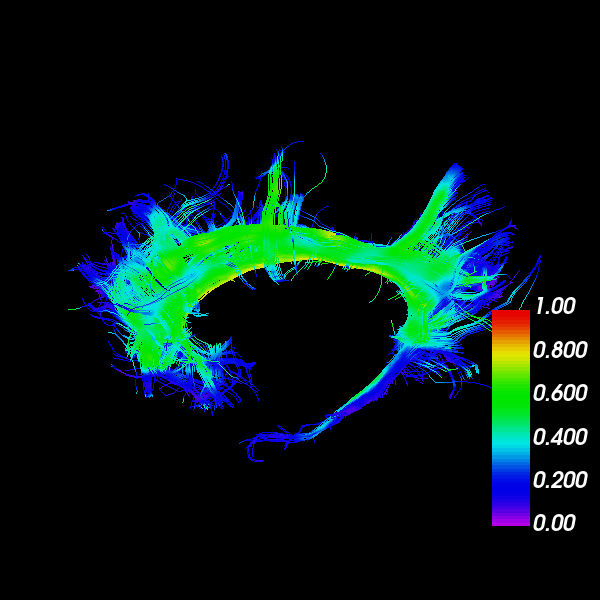## Show every point with a value from a volume with your colormap¶

Here we will need to input the `fa` map in `streamtube`

```scene.clear()

hue = (0.0, 0.0)  # red only
saturation = (0.0, 1.0)  # white to red

lut_cmap = actor.colormap_lookup_table(hue_range=hue,
saturation_range=saturation)

stream_actor3 = actor.line(bundle_native, fa, linewidth=0.1,
lookup_colormap=lut_cmap)
bar2 = actor.scalar_bar(lut_cmap)

if interactive:
window.show(scene, size=(600, 600), reset_camera=False)

window.record(scene, out_path='bundle3.png', size=(600, 600))
```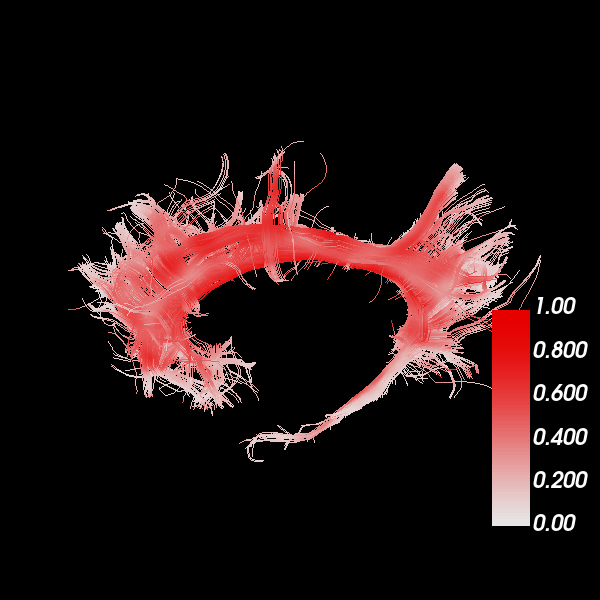## Show every bundle with a specific color¶

You can have a bundle with a specific color. In this example, we are chosing orange.

```scene.clear()
stream_actor4 = actor.line(bundle_native, (1., 0.5, 0), linewidth=0.1)

if interactive:
window.show(scene, size=(600, 600), reset_camera=False)

window.record(scene, out_path='bundle4.png', size=(600, 600))
```## Show every streamline of a bundle with a different color¶

Let’s make a colormap where every streamline of the bundle is colored by its length.

```scene.clear()

lengths = length(bundle_native)

hue = (0.5, 0.5)  # blue only
saturation = (0.0, 1.0)  # black to white

lut_cmap = actor.colormap_lookup_table(
scale_range=(lengths.min(), lengths.max()),
hue_range=hue,
saturation_range=saturation)

stream_actor5 = actor.line(bundle_native, lengths, linewidth=0.1,
lookup_colormap=lut_cmap)

bar3 = actor.scalar_bar(lut_cmap)

if interactive:
window.show(scene, size=(600, 600), reset_camera=False)

window.record(scene, out_path='bundle5.png', size=(600, 600))
```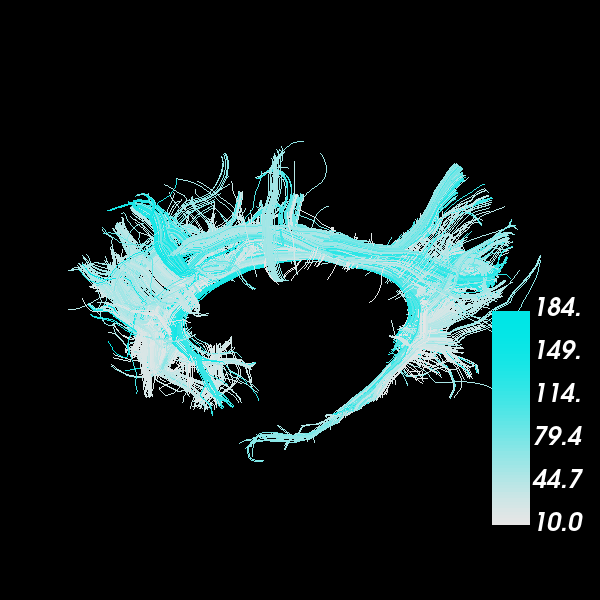## Show every point of every streamline with a different color¶

In this case in which we want to have a color per point and per streamline, we can create a list of the colors to correspond to the list of streamlines (bundles). Here in `colors` we will insert some random RGB colors.

```scene.clear()

colors = [np.random.rand(*streamline.shape) for streamline in bundle_native]

stream_actor6 = actor.line(bundle_native, colors, linewidth=0.2)

if interactive:
window.show(scene, size=(600, 600), reset_camera=False)

window.record(scene, out_path='bundle6.png', size=(600, 600))
```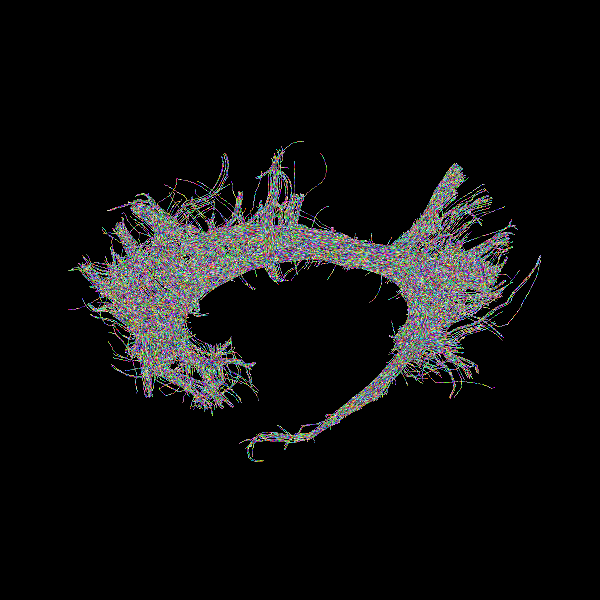## Add depth cues to streamline rendering¶

By default, lines are drawn with the same width on the screen, regardless of their distance from the camera. To increase realism, we can enable `depth_cue` to make the lines shrink with distance from the camera. We will return to the default color scheme from the first example. Note that `depth_cue` works best for `linewidth` <= 1.

```scene.clear()

stream_actor7 = actor.line(bundle_native, linewidth=0.5, depth_cue=True)

if interactive:
window.show(scene, size=(600, 600), reset_camera=False)

window.record(scene, out_path='bundle7.png', size=(600, 600))
```## Render streamlines as fake tubes¶

We can simulate the look of streamtubes by adding shading to streamlines with `fake_tube`. Note that `fake_tube` requires `linewidth` > 1.

```scene.clear()

stream_actor8 = actor.line(bundle_native, linewidth=3, fake_tube=True)

if interactive:
window.show(scene, size=(600, 600), reset_camera=False)

window.record(scene, out_path='bundle8.png', size=(600, 600))
```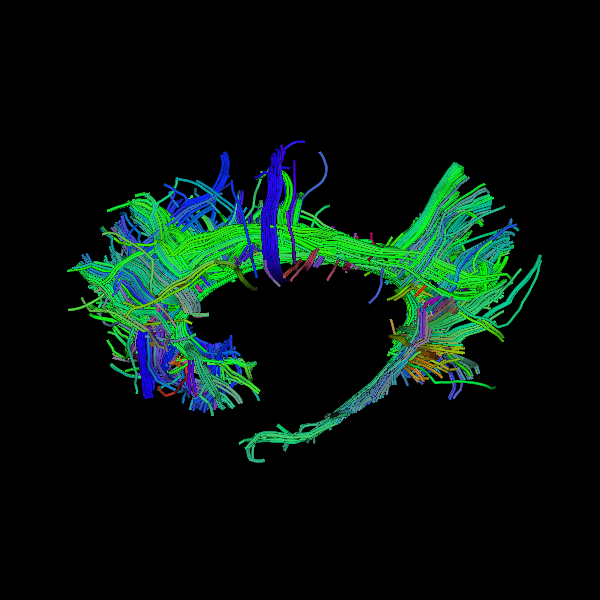## Combine depth cues with fake tubes¶

It is possible to fully simulate streamtubes by enabling both `depth_cue` and `fake_tube`. However, it can be challenging to choose a `linewidth` that demonstrates both techniques well.

```scene.clear()

stream_actor9 = actor.line(bundle_native, linewidth=3,
depth_cue=True, fake_tube=True)

if interactive:
window.show(scene, size=(600, 600), reset_camera=False)

window.record(scene, out_path='bundle9.png', size=(600, 600))
```## Render streamlines as tubes¶

For yet more realism, we can use `streamtube`. Note that this actor generates much more geometry than `line`, so it is more computationally expensive. For large datasets, it may be better to approximate tubes using the methods described above.

```scene.clear()

stream_actor10 = actor.streamtube(bundle_native, linewidth=0.5)